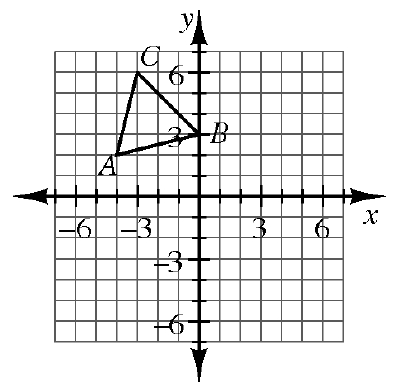### Home > CCG > Chapter 1 > Lesson 1.3.1 > Problem1-116

1-116.Copy $ΔABC$ at right on graph paper.

1. Rotate $ΔABC$ $90º$ counter-clockwise ($\circlearrowleft$) about the origin to create $ΔA'B'C'$. Name the coordinates of $C'$.

2. Reflect $ΔABC$ across the vertical line $x=1$ to create $ΔA''B''C''$. Name the coordinates of the vertices.

3. Translate $ΔABC$ so that $A'''$ is at $(4,-5)$. Name the coordinates of $B'''$.

See problem 1-60 and the Math Notes box for Lesson 1.2.2 (page 37 in the student text) for help with rotations.

Use the eTool below to view the rotations for parts (a), (b), and (c).
Click the link at right for the full version of the eTool: CCG 1-116 HW eTool (Desmos)